# Python implements ACO ant colony optimization algorithm to optimize Catboost regression model (CatBoostRegressor algorithm) project combat

Explanation: This is a machine learning practical project (with data + code + documentation + video explanation). If you need data + code + documentation + video explanation, you can go directly to the end of the article to get it.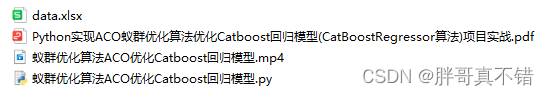## 1. Project background

Ant Colony Optimization (ACO) is a new bionic evolutionary algorithm derived from the natural biological world, adopted by Italian scholars M. Dorigo, V. Maniezzo and A. Colorni in the early 1990s. A population-based heuristic random search algorithm that simulates the collective path-finding behavior of ants in nature”. Ants have the ability to find the shortest path from the nest to the food source without any prompts, and can adapt to changes in the environment , adaptively search for new paths and produce new choices. The fundamental reason is that when ants are looking for food, they can release a special secretion-pheromone (also known as pheromone) on the path they have traveled. As time goes by, the substance will gradually volatilize, and the probability of subsequent ants choosing this path is proportional to the strength of the pheromone on this path at that time. When more and more ants pass through a path, the pheromone left by it There are more and more ants, and the probability of ants choosing this path is higher, thus increasing the pheromone intensity on this path. And the pheromone with high intensity will attract more ants, thus forming a positive feedback mechanism .Through this positive feedback mechanism, ants can finally find the shortest path.

This project uses the ACO ant colony optimization algorithm to find the optimal parameter value to optimize the Catboost regression model.

## 2. Data acquisition

The modeling data for this time comes from the Internet (compiled by the author of this project), and the statistics of the data items are as follows:The data details are as follows (partial display):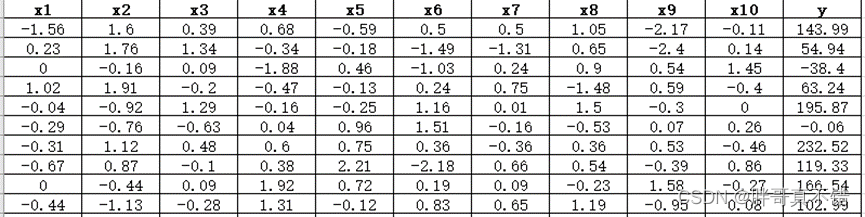## 3. Data preprocessing

### 3.1 View data with Pandas tools

Use the head() method of the Pandas tool to view the first five rows of data: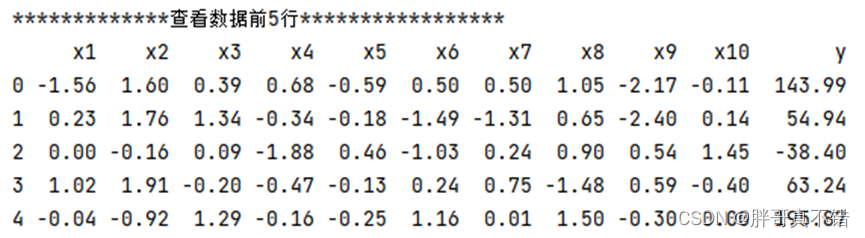key code:### 3.2 View missing data

Use the info() method of the Pandas tool to view data information: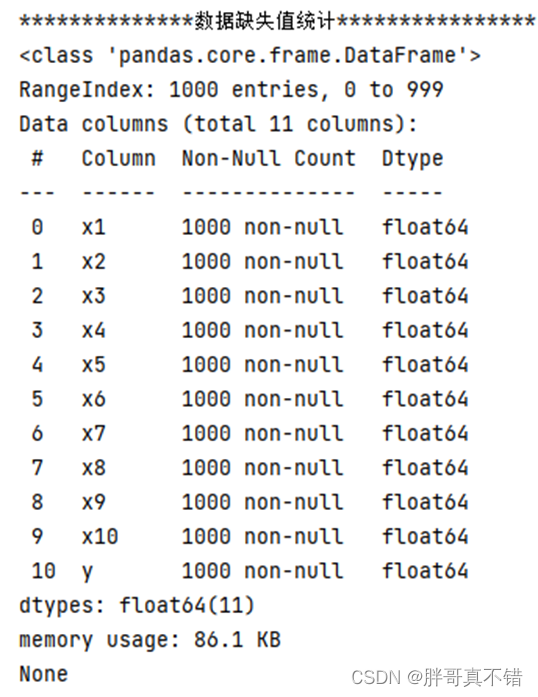As can be seen from the above figure, there are a total of 11 variables, and there are no missing values in the data, with a total of 1000 data.

key code: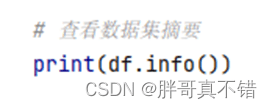### 3.3 Data descriptive statistics

Use the describe() method of the Pandas tool to view the mean, standard deviation, minimum, quantile, and maximum of the data.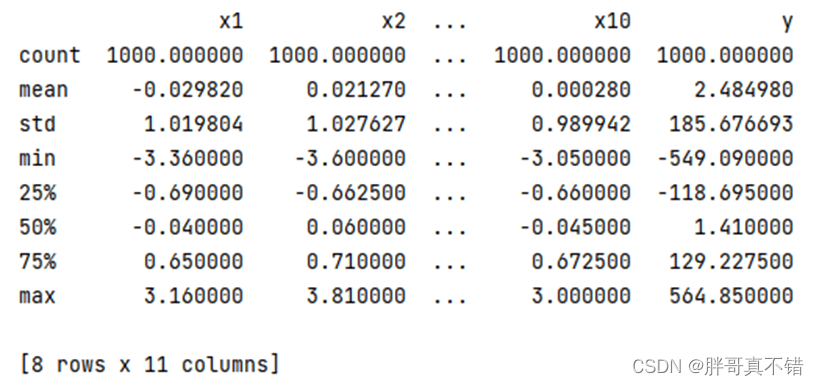The key code is as follows: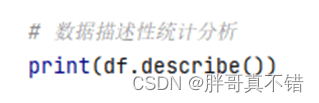## 4. Exploratory data analysis

### 4.1 y variable histogram

Use the hist() method of the Matplotlib tool to draw a histogram:As can be seen from the figure above, the y variable is mainly concentrated between -400 and 400.

### 4.2 Correlation Analysis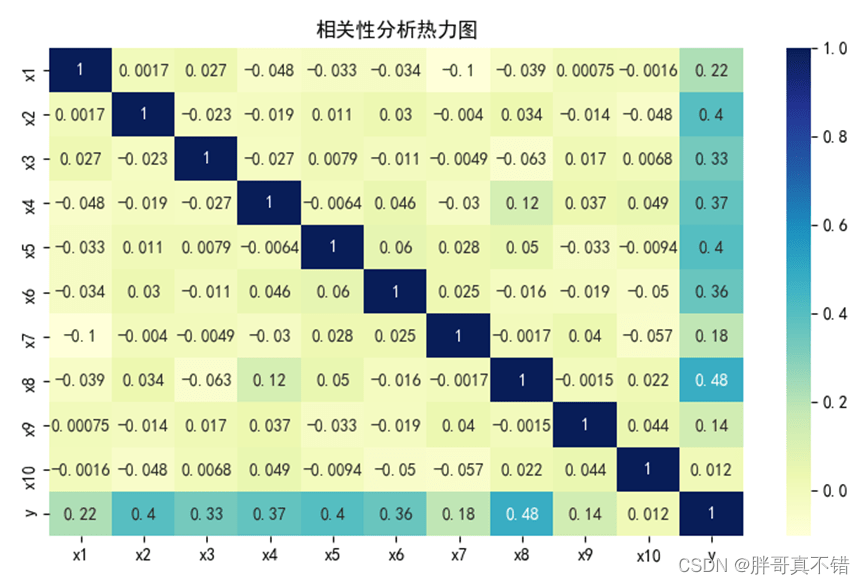As can be seen from the figure above, the larger the value, the stronger the correlation. A positive value is a positive correlation, and a negative value is a negative correlation.

## 5. Feature engineering

### 5.1 Create feature data and label data

The key code is as follows: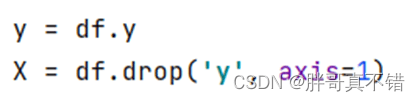### 5.2 Dataset Split

Use the train_test_split() method to divide according to 80% training set and 20% test set. The key code is as follows:## 6. Construct ACO ant colony optimization algorithm to optimize CATBOOST regression model

Mainly use the ACO ant colony optimization algorithm to optimize the CATBOOST regression algorithm for target classification.

### 6.1 Optimal parameters found by ACO ant colony optimization algorithm

key code: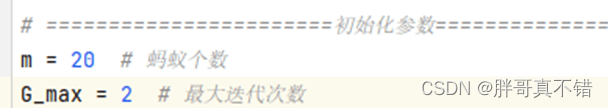Process data for each iteration: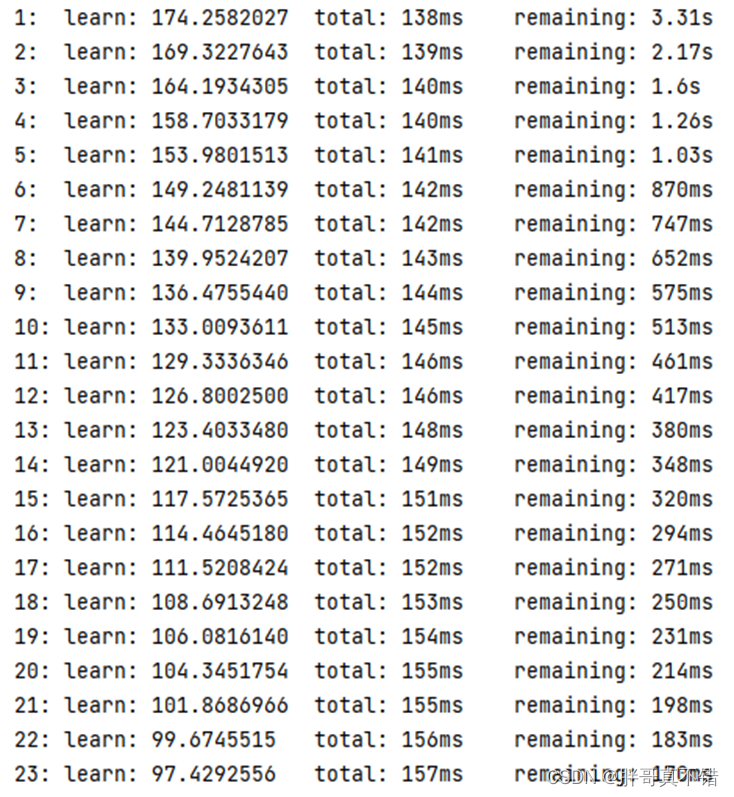optimal parameter:### 6.2 Optimal parameter value construction model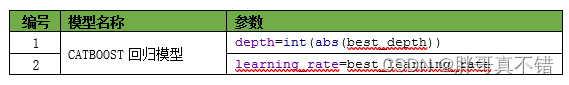## 7. Model evaluation

### 7.1 Evaluation indicators and results

The evaluation indicators mainly include explainable variance value, mean absolute error, mean square error, R square value and so on.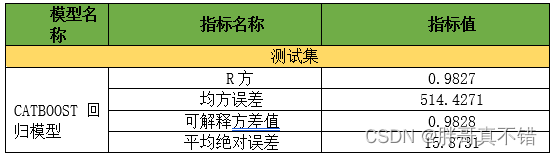It can be seen from the above table that the R square is 0.9827, which means that the model works well.

The key code is as follows:

###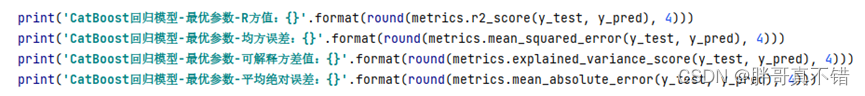7.2 Comparison chart of actual value and predicted value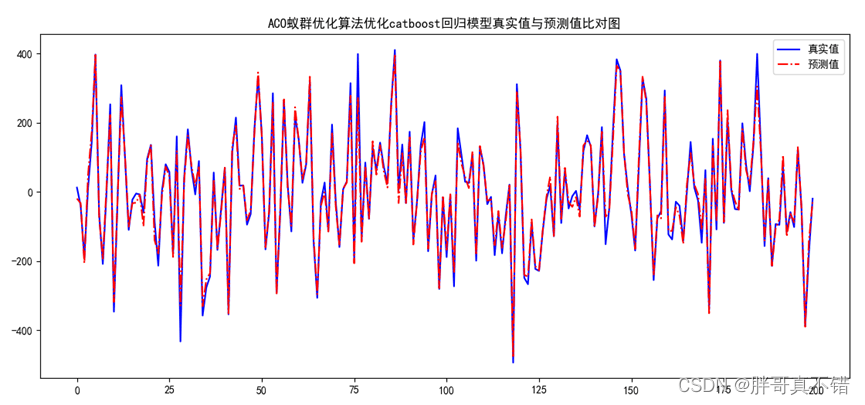It can be seen from the above figure that the fluctuations of the actual value and the predicted value are basically the same, and the model fitting effect is good.

## 8. Conclusion and prospect

To sum up, this paper uses the ACO ant colony optimization algorithm to find the optimal parameter values of the CATBOOST regression algorithm to build a regression model, which finally proves that the model we proposed works well. This model can be used for forecasting of everyday products.

```# ====Define penalty item function======
def calc_e(X):
"""Calculate the penalty item of ants, the dimension of X is size * 2 """
ee = 0
"""Calculate the penalty for the first constraint"""
e1 = X + X - 6
ee + = max(0, e1)
"""Calculate the penalty for the second constraint"""
e2 = 3 * X - 2 * X - 5
ee + = max(0, e2)
return ee

# *************************************************** *******************************

# The materials required for the actual combat of this machine learning project, the project resources are as follows:

# project instruction:

# Extract code: thgk

# *************************************************** *******************************

# ===Define the selection operation function between children and parents====
def update_best(parent, parent_fitness, parent_e, child, child_fitness, child_e, X_train, X_test, y_train, y_test):
"""
For different problems, the threshold of the penalty item is reasonably selected. In this example the threshold is 0.1
:param parent: parent individual
:param parent_fitness: parent fitness value
:param parent_e : parent penalty item
:param child: child individual
:param child_fitness child fitness value
:param child_e : child penalty item
:return: The better of parent and offspring, fitness, penalty
"""

if abs(parent) > 0: # Judgment value
depth = int(abs(parent)) + 3 # assignment
else:
depth = int(abs(parent)) + 5 # assignment

if abs(parent) > 0: # Judgment value
learning_rate = (int(abs(parent)) + 1) / 10 # assignment
else:
learning_rate = (int(abs(parent)) + 1) / 10 # assignment
```

For more project practice, see the list of machine learning project practice collections:

List of actual combat collections of machine learning projects